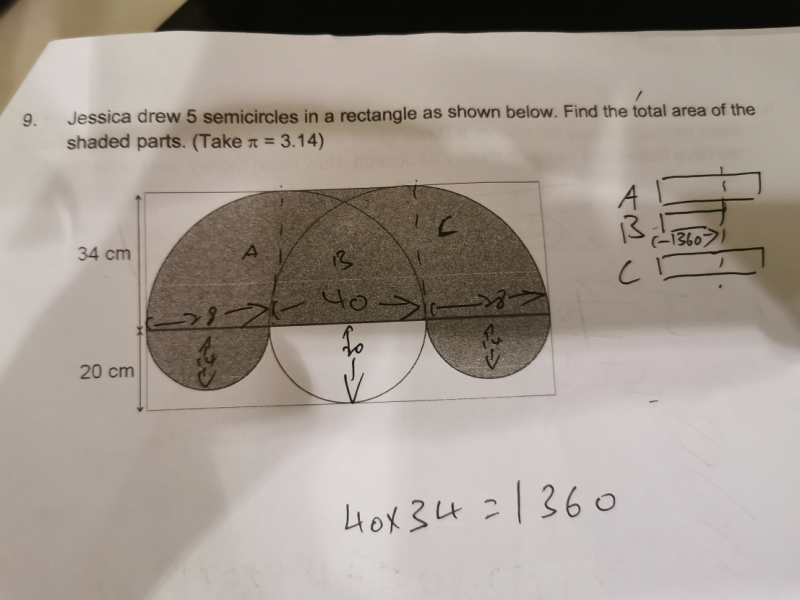# QuestionHi

please assist on the 2022 prelim question..

thanks

The 2 large semi-circles will meet the side of the rectangle at their highest point which is their radii.

So the rectangle between them would have a breadth = (28+28+40) – 34 – 34 = 28

So Area of shaded part

= Area of 1 large semi circle + Area of rectangle between the large semi circles + Area of 1 small circle

= (1/2)(3.14)(34)2 + (34)(28) + (3.14)(14)2

= 1814.92 + 952 + 615.44

= 3382.36

Hence the area of shaded part is 3382.36cm2

0 Replies 2 Likes